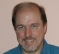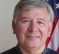## harmonic distortion of a single-tone excitationStarted by 6 years ago6 replieslatest reply 6 years ago259 views

I consider a single-tone input to a nonlinear amplifier. I know, the nonlinearity will produce additional harmonic components at the output. I also know, the amplitude of the harmonic components will be smaller than the amplitude of the fundamental component. But will the phase be different as well?

So, if x(t) is the input signal, is y(t) a valid description of the output signal?

x(t) = A1 * sin(2*pi*f1*t + Phi1)

y(t) = sum( A_n * sin(2*pi*n*f1*t + Phi_n) )

[ - ]I believe the answer to your first question is Yes. Let's assume your Phi1 = p and w1 = 2*pi*f1. If your input is:

x(t) = A1*sin(w1*t + p),

the output of a slightly nonlinear amplifier is modeled as (assumed to be reasonably accurately represented by):

y(t) = B1*x(t) + B2*[x(t)^2]

= B1*A1*sin(w1*t + p) + B2*A1^2*[sin(w1*t + p)^2].

Using a trig identity for sine squared we can write:

y(t) = B1*A1*sin(w1*t + p)

+ (B2*A1^2)/2 -[(B2*A1^2)/2]*cos(2w1*t + 2p).

Your nonlinear amplifier's output should be a DC component, plus your original f1 Hz sine wave spectral component with a phase of Phi1, and a 2f1 Hz negative-cosine spectral component whose phase is 2Phi1. I suggest you double check the validity of my comments using software.

[ - ]allright, thx!

I don't have software to validate your answer, but it is what I figured.

[ - ]"I also know, the amplitude of the harmonic components will be smaller than the amplitude of the fundamental component."

Build an amplifier with two amplifying elements, biased for class B or C, and driven 180 degrees out of phase.  Tie their anodes (collectors, drains, plates, whatever) together.

It's called a push-push amplifier, and if it is designed and built well, the fundamental and all of the odd harmonics are significantly suppressed.  It's a very handy thing for frequency multiplication, particularly doubling.

[ - ]The amplitudes of the harmonic-distortion components are smaller than the fundamental amplitude for a "weak nonlinearity", but not in general.  Consider the squaring nonlinearity - no fundamental at all - just dc plus an octave up.   Your output Fourier series expansion looks correct if n starts at 0 and goes to infinity.  It's hard to say anything general about the phases when the nonlinearity can be anything.  Consider the nonlinear system that first clips the input sinusoid to create an infinite row of harmonics, and then rotates each harmonic phase by an arbitrary signal-dependent angle, and reconstructs.  You can have any phase you want for each harmonic.  Add some delay and you get any phase for the fundamental as well.
[ - ]I think you can easily get the answer by applying Fourier series analysis on the output (nonlinear) signal.

[ - ]Since the component frequencies are different but harmonically related, the phase components will be related but can't be the "same".  Consider that a phase shift of a sinusoid is equivalent to a time delay.

The waveform in the case you've given is static - i.e. the waveshape is always the same.  This means that the relative time delay of the harmonics is smaller but aligned with the fundamental.  We don't normally refer to phase being "423 degrees", eh?

Also consider that the "amplifier" could well have frequency dependencies.  It's a filter.  This will modify the phase of various output components.  while their relative alignment in time will be stable, it could be anything within reason.

So yes the phase will be different (if that has meaning) as well.  Just make sure the meaning is clear.  Here's an example:

First and 3rd harmonics at the output only:

Use the first harmonic positive-going axis crossing as a time reference.

The 3rd harmonic next positive going axis crossing happens after some fraction of the 3rd harmonic period which is also 1/3 the value of the fraction of the fundamental period.  Either one could be used to state a "phase" value - but those values are different by a factor of 3.  It's probably better to use time delay so there's no ambiguity - at least in forming a mental picture of it.

Of course, the Fourier Series does this and expresses things in phase but it's always relative to each harmonic.  So that's a good definition for it.